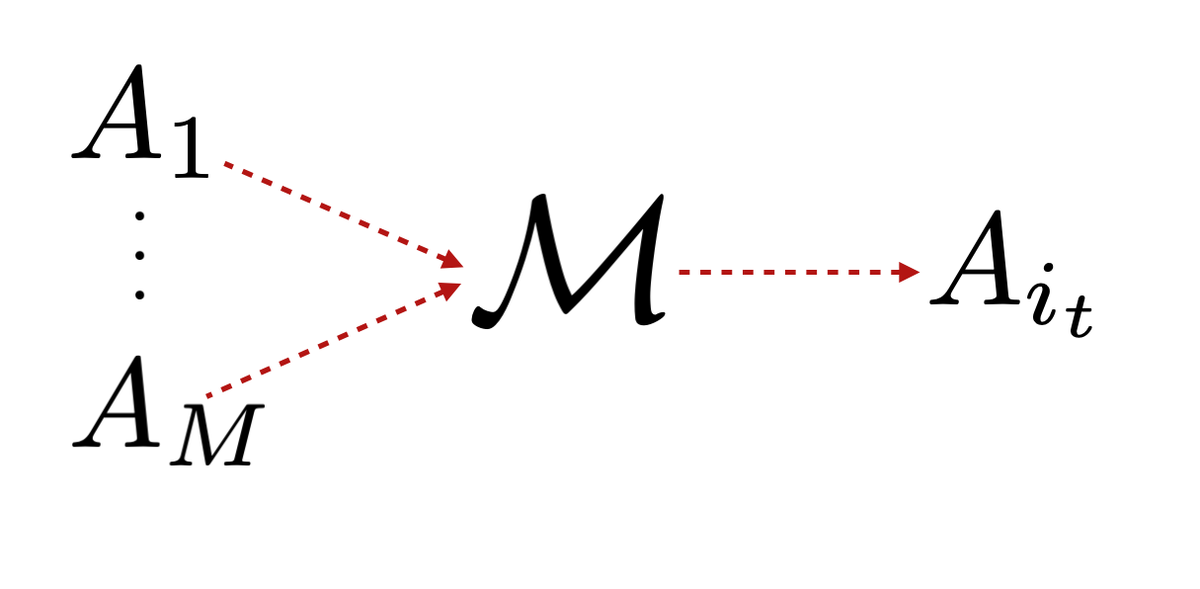# Model Selection for Contextual Bandits and Reinforcement Learning

From Stochastic CORRAL to Regret Balancing.## Overview

In many domains ranging from internet commerce, to robotics and computational biology, many algorithms have been developed that make decisions with the objective of maximizing a reward, while learning how to make better decisions in the future. In hopes of realizing this objective a vast literature focused on the study of Bandits and Reinforcement Learning algorithms has arisen. Although in most practical applications, precise knowledge of the nature of the problem faced by the learner may not be known in advance most of this work has chiefly focused on designing algorithms with provable regret guarantees that work under specific modeling assumptions. Less work has been spent on the problem of model selection where the objective is to design algorithms that can select in an online fashion the best suitable algorithm among a set of candidates to deal with a specific problem instance.

## Papers

• Model Selection in Contextual Stochastic Bandit Problems - In this work we introduce the Stochastic CORRAL algorithm for model selection in stochastic contextual bandit problems and RL. The Stochastic CORRAL algorithm makes use of a master algorithm based on the CORRAL master. We also show some initial lower bounds showing the imposibility of deriving model selection results in a variety of settings.
• Online Model Selection for Reinforcement Learning with Function Approximation - We introduce the Explore-Commit-Eliminate (ECE) algorithm for model selection. This algorithm is suitable for model selection between stochastic bandit and reinforcement learning algorithms. ECE is based on a simple misspecification test coupled with a play schedule reminiscent of epsilon greedy algorithms.
• Regret Bound Balancing and Elimination for Model Selection in Bandits and RL - This work proposes the Regret Bound Balancing and Elimination Algorithm (RBBE). This algorithm can be used for model selection among stochastic bandit and Reinforcement Learning algorithms. Similar to the ECE algorithm, RBBE is based on a simple misspecification test, in this case coupled with an algorithm play schedule based on balancing the base learner’s regret bounds. Additionally, we show this approach can be extended to the setting of adversarially generated contexts in stochastic contextual linear bandits.
• Dynamic Balancing for Model Selection in Bandits and RL - This work extends and refines the RBBE approach, shaving polynomial factors in the number of models from the resulting model selection regret upper bounds.
• Model Selection for Contextual Bandits and Reinforcement Learning - PhD Thesis. A compendium of the results introduced above. -Best of Both Worlds Model Selection - This works extends the regret balancing technology to work for adversarial bandits. It also explores the question of wether it is possible to obtain model selection and best of both worlds guarantees between adversarial and stochastic bandit scenarios.##### Aldo Pacchiano
###### Postdoctoral Researcher

My research interests include online learning, Reinforcement Learning, Deep RL and Fairness.